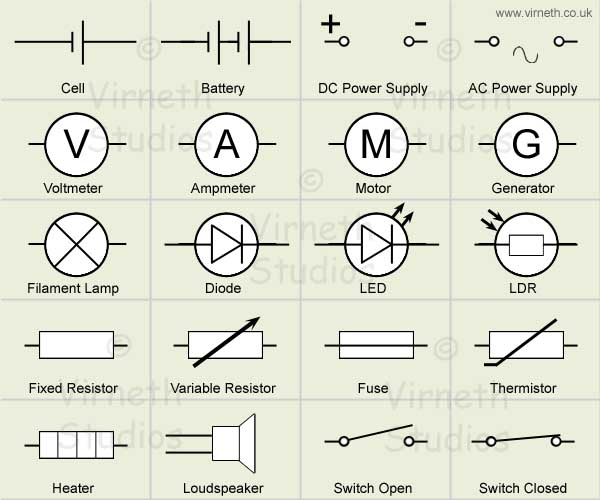# Electric Circuits

Electricity needs a complete continuous circuit to flow. There are two basic types of circuit: Series and Parallel.

## Series Circuits

In a Series circuit all components are connected one after another in a line.

• If you remove any component then the circuit is broken
• The total resistance of the circuit is the sum of the resistances of all the components
• Every component has the same current running through it
• The current is calculated by dividing the total potential difference by the total resistance of the circuit I=V/R
• The voltages of all the components add up to the total voltage of the supply
• The bigger the resistance of a component, the bigger its share of the p.d. (voltage)

Series circuits are not very common because the whole circuit fails when one component fails.

## Parallel Circuits

In a parallel circuit the +ve ends of two or more components all share the same connection and the –ve ends all share another common connection.

• If you remove one component, the others still work
• The voltage is the same for all the components
• The current through a component is inversely proportional to the resistance. This means that bigger the resistance the lower the current.
• Total current is equal to the sum of all the currents in each branch. The current is shared out amongst all the branches

## Components

If a circuit is to be useful it must include components. In a circuit diagram these components are represented by symbols. All circuits must have some sort of power supply, so battery and generator symbols are used regularly. Other symbols that are common include lights, switches and resistors. Complex circuits will include transistors, semiconductors, diodes and microchips amongst others. Microchips are actually complete miniature circuits in themselves etched onto a piece of silicon, they will often have their own circuit diagram and allow engineers to design circuits using modular components that are built and tested independently of the main design.

Here are some of the more common symbols:Common symbols include a cell, a battery, switches, meters, power supplys, motors, heaters and others